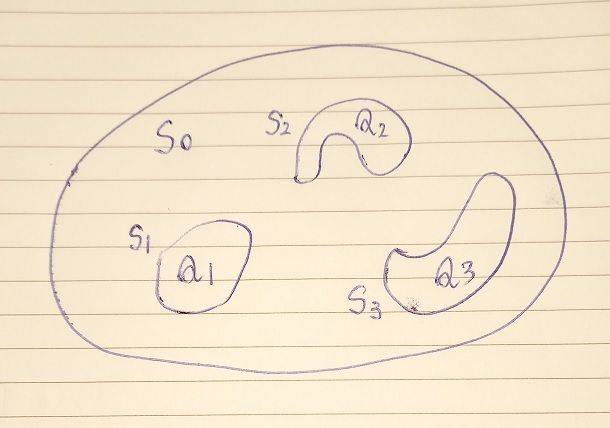# The second uniqueness theorem in electrostatics

• I
Ahmed1029
Does the second uniqueness theorem just say that if there is an electric field that satisfies Gauss's law for a surface surrounding each conductor + a surface enclosing all the conductors, it is indeed the true electric field, and no other electric field will satisfy those conditions?

alan123hk
In the link below, the second uniqueness theorem is described as follows.

"The second uniqueness theorem states that the electric field is uniquely determined if the total charge on each conductor is given and the charge distribution in the regions between the conductors is known"

http://teacher.pas.rochester.edu/PHY217/LectureNotes/Chapter3/LectureNotesChapter3.html

I think this conclusion clearly makes sense even without a rigorous mathematical proof.

Last edited:
•vanhees71
Ahmed1029
"The second uniqueness theorem states that the electric field is uniquely determined if the total charge on each conductor is given and the charge distribution in the regions between the conductors is known"
I know, but I don't know how to use it when stated like this.
Is what I described above the right way to use it?

alan123hk
I think it's a little different from what you said.

In the figure below, the respective total charges q1, q2, and q3 of conductors s1, s2, and s3 are known, but the charge distribution on each conductor (S1, S2 and S3) is unknown.

Furthermore, the exact charge distribution in all other spaces S0 containing these three conductors is also known.Ahmed1029
I think it's a little different from what you said.

In the figure below, the respective total charges q1, q2, and q3 of conductors s1, s2, and s3 are known, but the charge distribution on each conductor (S1, S2 and S3) is unknown.

Furthermore, the exact charge distribution in all other spaces S0 containing these three conductors is also known.

that doesn't tell me how to use it or what conditions the electric field must satisfy to be true. All it says is that there is one electric field that is true, it doesn't tell me how to know which one is true. For example I give you 5 different electric fields and ask you which one is the true one, what will you do? What conditions are you going to apply ?

alan123hk
My understanding is that the uniqueness theorem only tells us that the electric field is unique and there cannot be another different electric field. So it can't tell us which electric field is true.

If we want to know what this unique electric field looks like, we must know the exact distribution of charges on conductors s1,s2 and s3.

•vanhees71 and Ahmed1029